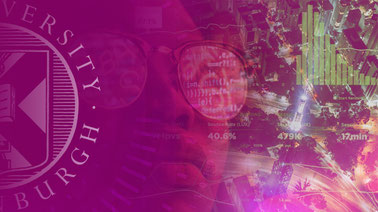## Monday, July 27, 2020

### Statistical Predictive ModellingIn this course, you&nbsp;will learn about two key predictive modelling techniques: linear and logistic regression, and look at several&nbsp;relevant applications to see how they can be used in real-world scenarios.Before delving into advanced modelling methods,&nbsp;you will benefit from&nbsp;gaining a solid understanding of these two basic modelling techniques. They are the workhorse of effective predictive modelling, based on whether&nbsp;a response is continuous or categorical. If you take this course as part of the MicroMasters programme, you will&nbsp;discover that many advanced techniques are&nbsp;actually adjustments to linear (logistic) regression.The first half of the course focuses on linear regression. This technique allows you to model a continuous outcome variable using both continuous and categorical predictors. This enables you, for example, to predict product sales based on a number of customer variables.In the second half of the course, you will learn about logistic regression, which is the counterpart of linear regression, when the response variable is categorical. You will also be introduced to na&iuml;ve Bayes; a very intuitive, probabilistic modelling technique.